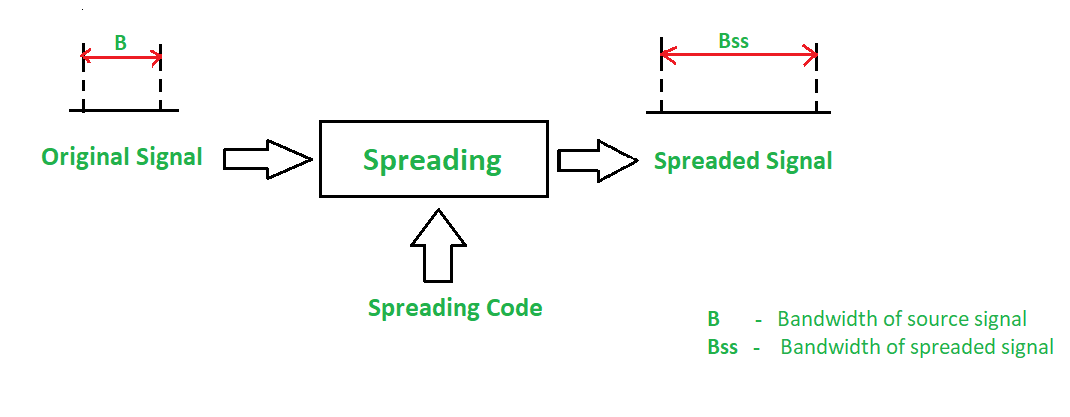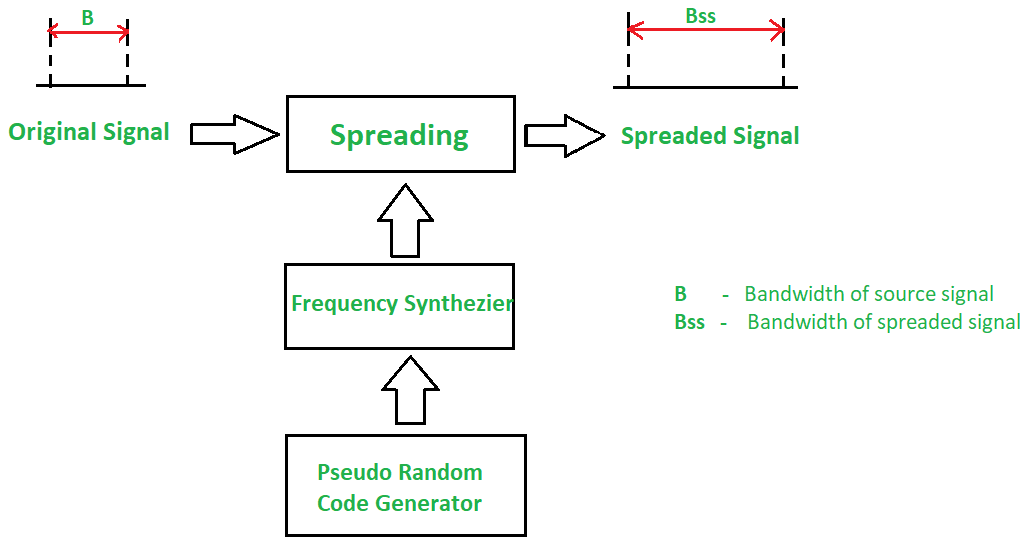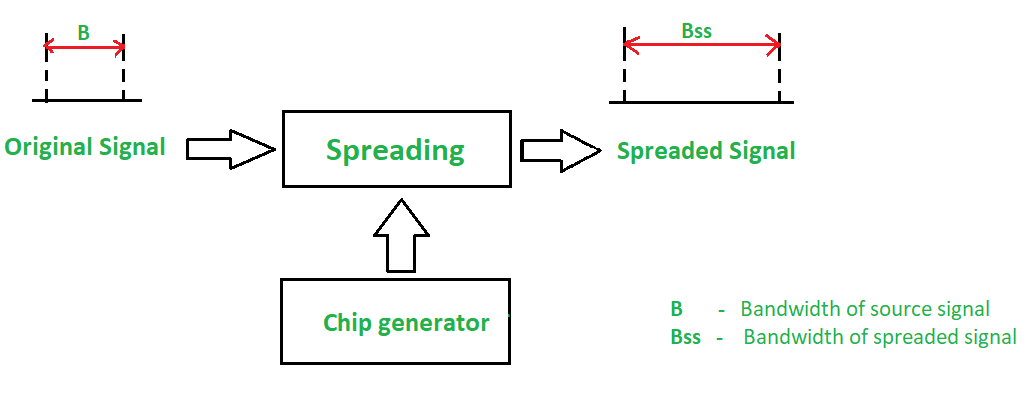Open In App

The increasing demand for wireless communications has problems due to limited spectrum efficiency and multipath propagation. The use of spread spectrum communication has simplified these problems. In the spread spectrum, signals from different sources are combined to fit into larger bandwidth

Most stations use air as the medium for communication, stations must be able to share the medium without an interception and without being subject to jamming from a malicious intruder. To achieve this, spread-spectrum techniques add redundancy means it uses extended bandwidth to accommodate signals in a protective envelope so that more secure transmission is possible. The spread code is a series of numbers that looks random but are actually a pattern. The original bandwidth of the signal gets enlarged (spread) through the spread code as shown in the figure.### Principles of Spread Spectrum process:

1. To allow redundancy, it is necessary that the bandwidth allocated to each station should be much larger than needed.
2. The spreading process occurs after the signal is created by the source.

### Conditions of Spread Spectrum are:

1. The spread spectrum is a type of modulation where modulated signal BW is much larger than the baseband signal BW i.e. spread spectrum is a wide band scheme.
2. A special code (pseudo noise) is used for spectrum spreading and the same code is to be used to despread the signal at the receiver.

### Characteristics of the Spread Spectrum are:

1. Higher channel capacity.
2. Ability to resist multipath propagation.
3. They cannot easily intercept any unauthorized person.
4. They are resistant to jamming.
5. The spread spectrum provides immunity to distortion due to multipath propagation.
6. The spread spectrum offers multiple access capabilities.

### Two types of techniques for Spread Spectrum are:

1. Frequency Hopping Spread Spectrum (FHSS)
2. Direct Sequence Spread Spectrum (DSSS)

### Frequency Hopping Spread Spectrum (FHSS):

In Frequency Hopping Spread Spectrum (FHSS), different carrier frequencies are modulated by the source signal i.e. M carrier frequencies are modulated by the signal. At one moment signal modulates one carrier frequency and at the subsequent moments, it modulates other carrier frequencies. The general block diagram of FHSS is shown in the below figure.A pseudorandom code generator generates Pseudo-random Noise of some pattern for each hopping period Th. The frequency corresponding to the pattern is used for the hopping period and is passed to the frequency synthesizer. The synthesizer generates a carrier signal of that frequency. The figure above shows the spread signal via FHSS.

• Synchronization is not greatly dependent on distance.
• Processing Gain is higher than DSSS.

• The bandwidth of the FHSS system is too large (in GHz).
• Complex and expensive Digital frequency synthesizers are required.

### Direct Sequence Spread Spectrum (DSSS):

In DSSS, the bandwidth of the original signal is also expanded by a different technique. Here, each data bit is replaced with n bits using a spreading code called chips, and the bit rate of the chip is called as chip-rate. The chip rate is n times the bit rate of the original signal. The below Figure shows the DSSS block diagram.In wireless LAN, the sequence with n = 11 is used. The original data is multiplied by chips (spreading code) to get the spread signal. The required bandwidth of the spread signal is 11 times larger than the bandwidth of the original signal.